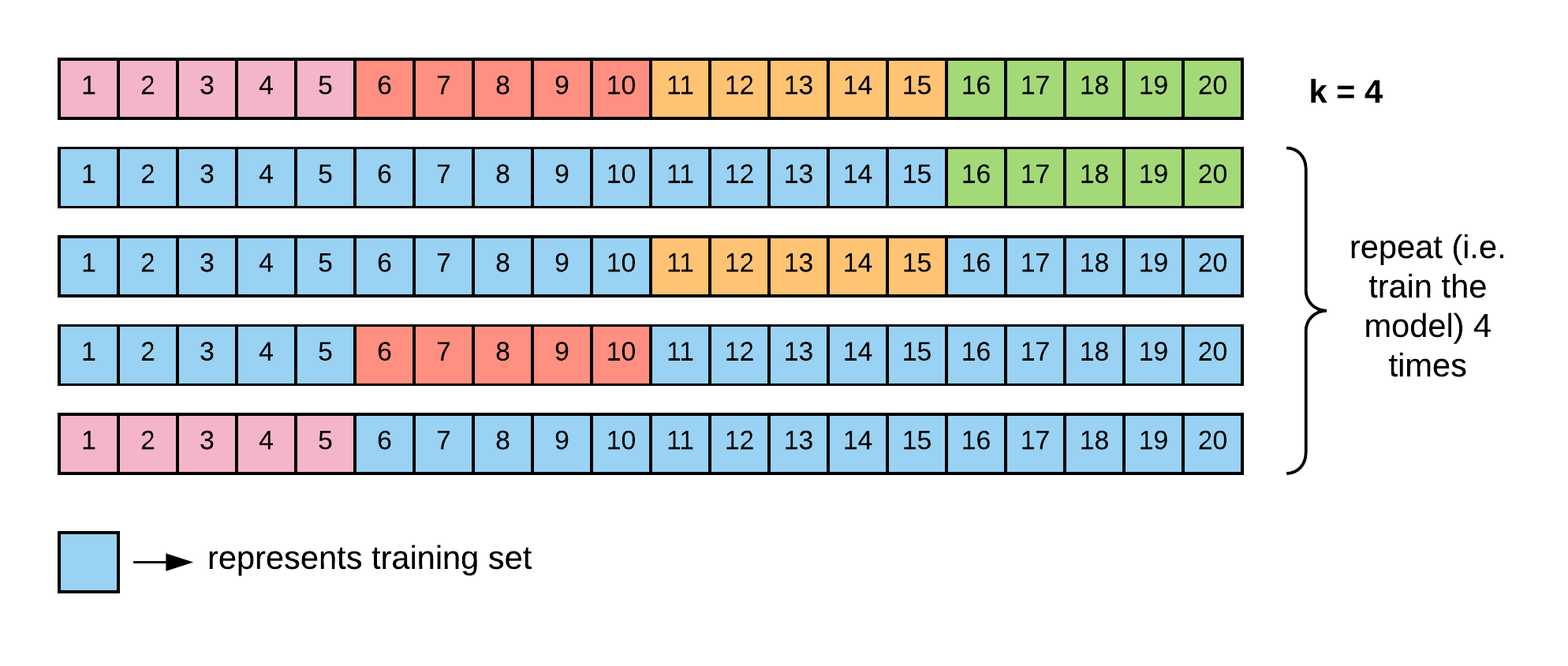Goal: Teach the machine to learn based on the input they receive from the Environment.

Tom Mitchell (1997) defines machine learning as the ability for a machine to gain expertise on a particular task based on experience.

## Why Machine Learning?

• Solving complexity: Excels in building computational engines to solve task that cannot possibly be explicitly programmed.
• Examples include natural language understanding, speech recognition and facial recognition.

## Types of Learning

Machine Learning can be categorized into (at least) three components based on its approach.

The three predominant schemes of learning are:

• Supervised,
• Unsupervised, and
• Reinforcement Learning

Supervised Learning

• Each data point is associated with a label
• Goal: Teach the computer using this labeled data.
• Learning: The computer learns the patterns from data.
• Inference: Makes decisions about “unknown” samples.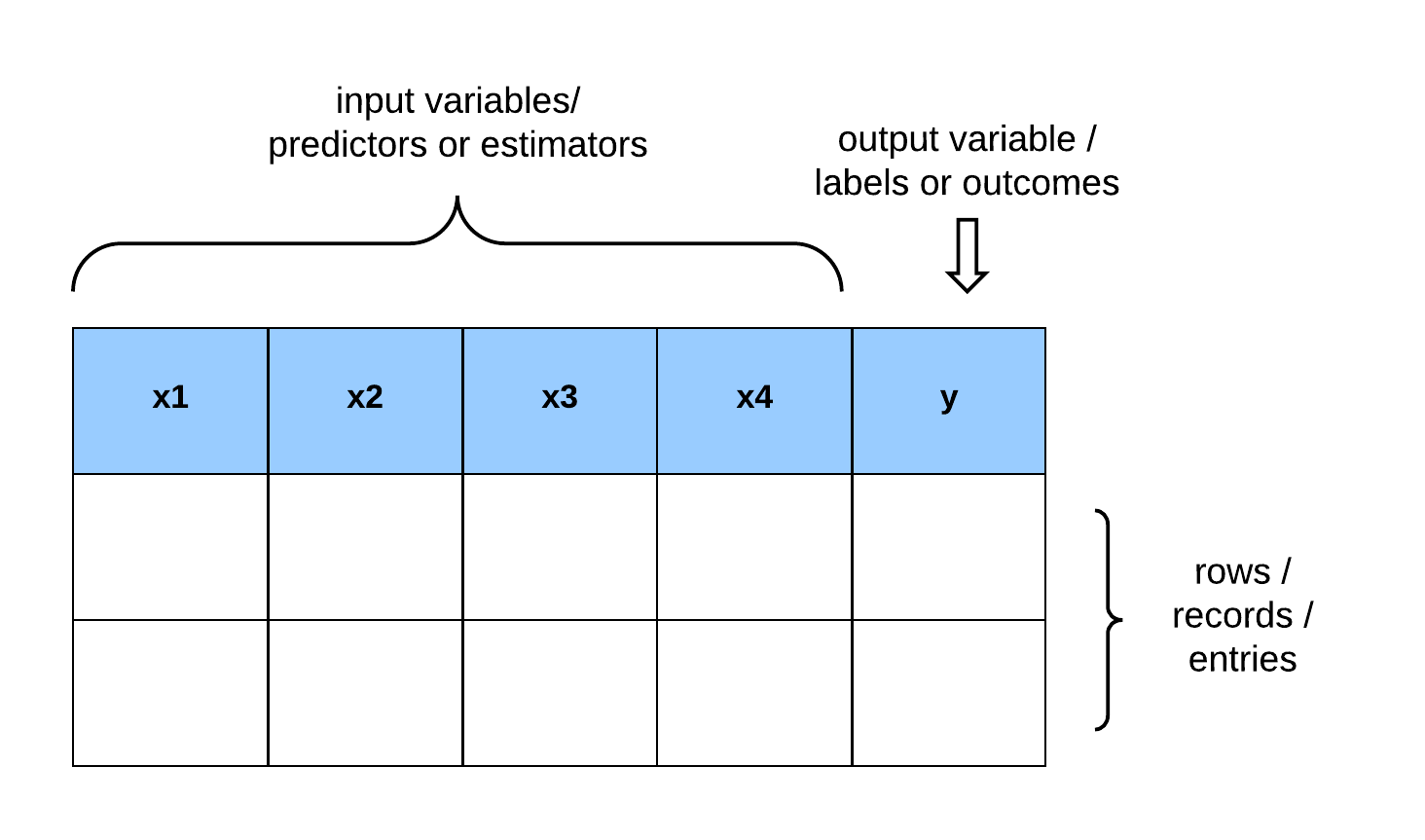Unsupervised Learning

• No corresponding labels - no guidance.
• Goal: Computer attempts to determine data’s unknown structure.
• Scheme: By “grouping” similar samples together adaptively.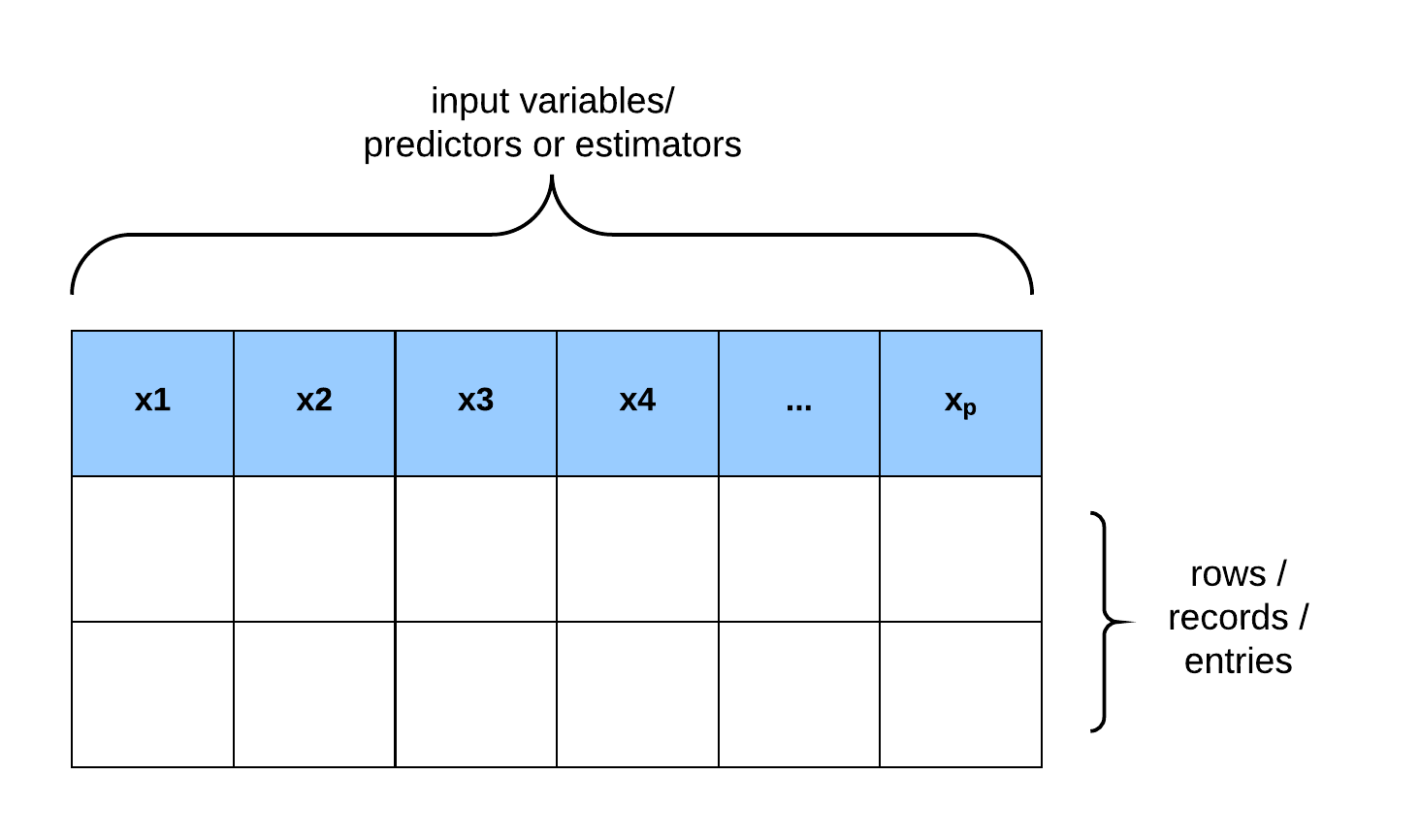Reinforcement Learning

• Reinforcement Learning: Agent interacts with an Environment.
• Scheme: A “feedback configuration”.
• Method: Chooses an action from the set of actions.
• Learning: Based on the responses from the Environment.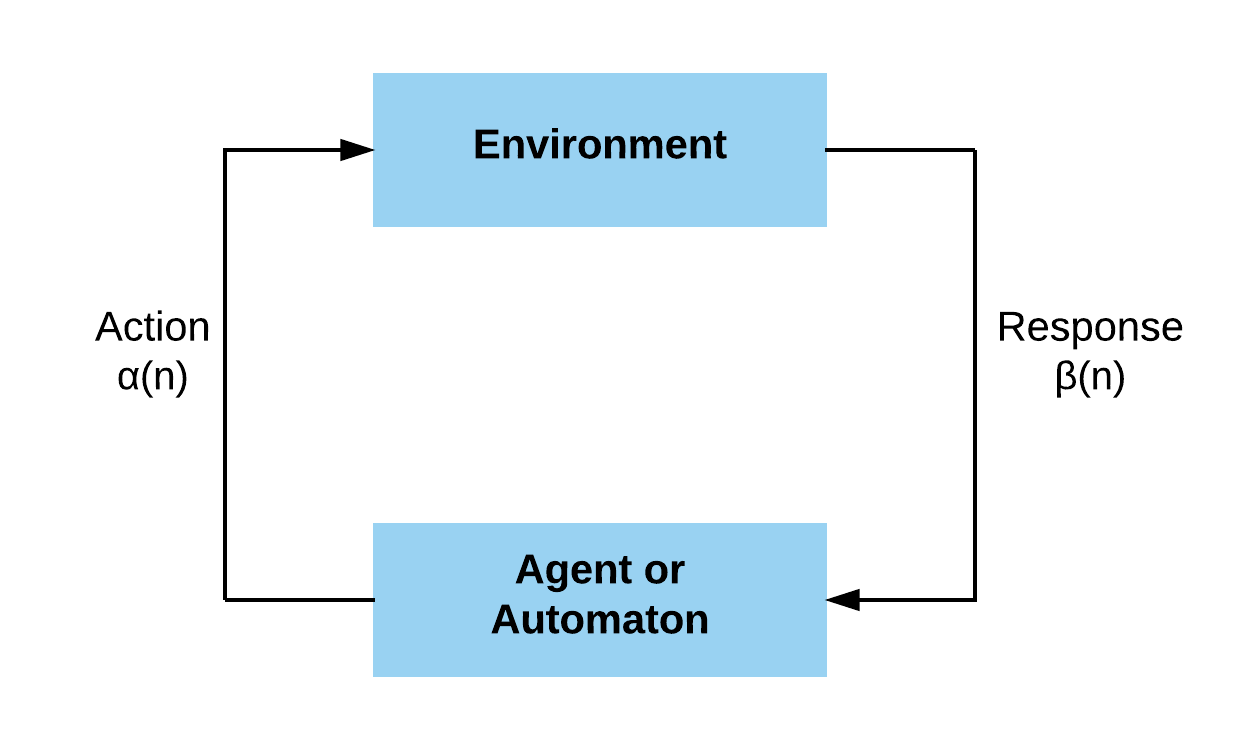## Foundations of Machine Learning

The foundational disciplines that contribute to the field of machine learning are:

• Statistics,
• Mathematics,
• The Theory of Computation, and to a considerable extent
• Behavioral Psychology

The diagram below visually shows the interaction between these fields.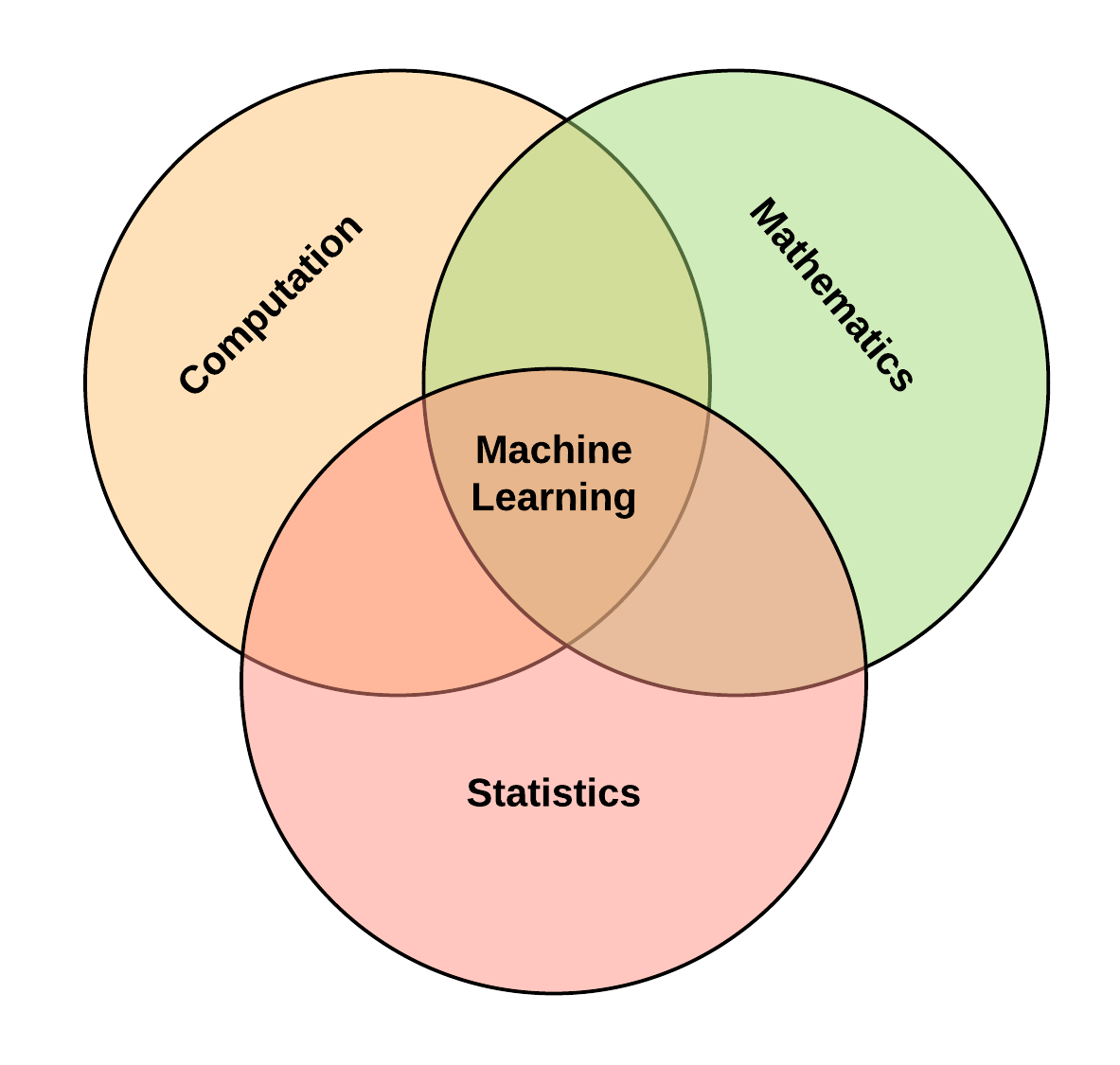## A Formal Model for Machine Learning Theory

The learner’s input:

• Domain set: An arbitrary set $\mathcal{X}$ that characterizes a set of objects that we want to label. The domain points are represented as a vector of features and are also called instances, with $\mathcal{X}$ the instance space.
• Label set: Let $\mathcal{Y}$ denote our set of possible labels. For example, let the label set be restricted to a two-element set, {0, 1} or {−1, +1}.
• Training data: $S = ((x_1, y_1)...(x_m, y_m))$ is a finite sequence of pairs in $\mathcal{X} \times \mathcal{Y}$ (i.e. a sequence of labeled domain points). The training data is the input that the learner has access to. $S$ is referred to as a training set.

The learner’s output: The learner returns a prediction rule, $h : \mathcal{X} \rightarrow \mathcal{Y}$. This function is also called a predictor, a hypothesis, or a classifier. The predictor is used to predict the label of new domain points. The notation $A(S)$ denotes the hypothesis that an algorithm, $A$, outputs, operating on the training set $S$.

The data generating model: The training set are generated by some probability distribution that is “unknown” to the learner. The probability distribution over $\mathcal{X}$ is denoted by $\mathcal{D}$. In instances where the correct labelling is known, then each pair in the training data $\mathcal{S}$ is generated by first sampling a point $x_i$ according to $\mathcal{D}$ and then labeling it by $f$, where, $\;f : \mathcal{X} \rightarrow \mathcal{Y}$, and that $y_i = f(x_i)$ for all $i$.

Measuring success: The error of a classifier is the probability that it does not predict the correct label on a random data point generated by the underlying distribution. Therefore, the error of $h$ is the probability to draw a random instance $x$, according to the distribution $\mathcal{D}$, such that $h(x)$ does not equal $f(x)$.

The error of the prediction rule such $\;h : \mathcal{X} \rightarrow \mathcal{Y}\;$ is the probability of randomly choosing an example $x$ for which $h(x) \neq f(x)$:

where,

• The subscript $(\mathcal{D}, f)$: indicates that the error is measured with respect to the probability distribution \mathcal{D} and the correct labeling function $f$.
• $L_{\mathcal{D},\;f} (h)$: is the generalization error, the risk, or the true error of $h$.

Note: The learner is blind to the underlying distribution $\mathcal{D}$ over the world and to the labeling function $f$.

### Empirical Risk Minimization

<img src="/assets/ieee_ompi/learning_model.png" alt="Learning Model."height="80%" width="80%"/>

The goal of the learning algorithm is to find the $h_S$ that minimizes the error with respect to the unknown $\mathcal{D}$ and $f$. Since the learner is unaware of $\mathcal{D}$ and $f$, the true error is not directly available to the learner.

The training error (also called empirical error or empirical risk) is the error the classifier incurs over the training sample:

\begin{equation} L_S(h) = \frac{|{i \in [m] : h(x_i) \neq y_i}|} {m} \end{equation}

where,

• [m] is the total number of training examples.

The hypothesis $h$ that minimizes the error $L_S(h)$ is called Empirical Risk Minimization or ERM.

## How do we assess learning?

Assume a teacher teaches a physics class for three months, and at the end of the lecture sessions, the teacher administers a test to ascertain if the student has learned.

Let us consider two different sub-plots:

1. The teacher tests the student with an exact word for word replica of questions that he used as examples while teaching.
2. The teacher evaluates the student with an entirely different but similar problem set based on the principles taught in class. In which of the subplots can the teacher be confident that the student has learned?

### Norms of Learning

1. Memorization: Memorization is the act of mastering and storing a pattern for future recollection. Therefore it is inaccurate to use training samples to carry out learning evaluation. In machine learning, this is also known as data snooping.
2. Generalization: The ability for the student to extrapolate using the principles taught in class to solve new examples is known as Generalization. Hence, we can conclude that learning is the ability to generalize to new cases.

## Training and Validation data

Again, the goal of machine learning is to predict or classify outcomes on unseen observations. Hence, the dataset is partitioned into:

• Training set: for training the model.
• Validation set: for fine-tuning the model parameters.
• Test set: for assessing the true model performance on unseen examples.

A common strategy is to split 60\% of the dataset for training, 20% for validation, and the remaining 20% for testing. This is commonly known as the 60/20/20 rule.

<img src="/assets/ieee_ompi/train-test-validation-set.png" alt="Dataset partitions."height="60%" width="60%"/>

• The bias/ variance tradeoff is critical for assessing the performance of the machine learning model.
• Bias is when the learning algorithm fails oversimplifies the learning problem and fails to generalize to new examples.
• Variance is when the model “closely” learns the irreducible error of the dataset. This leads to high variability in the presence of an unseen observation.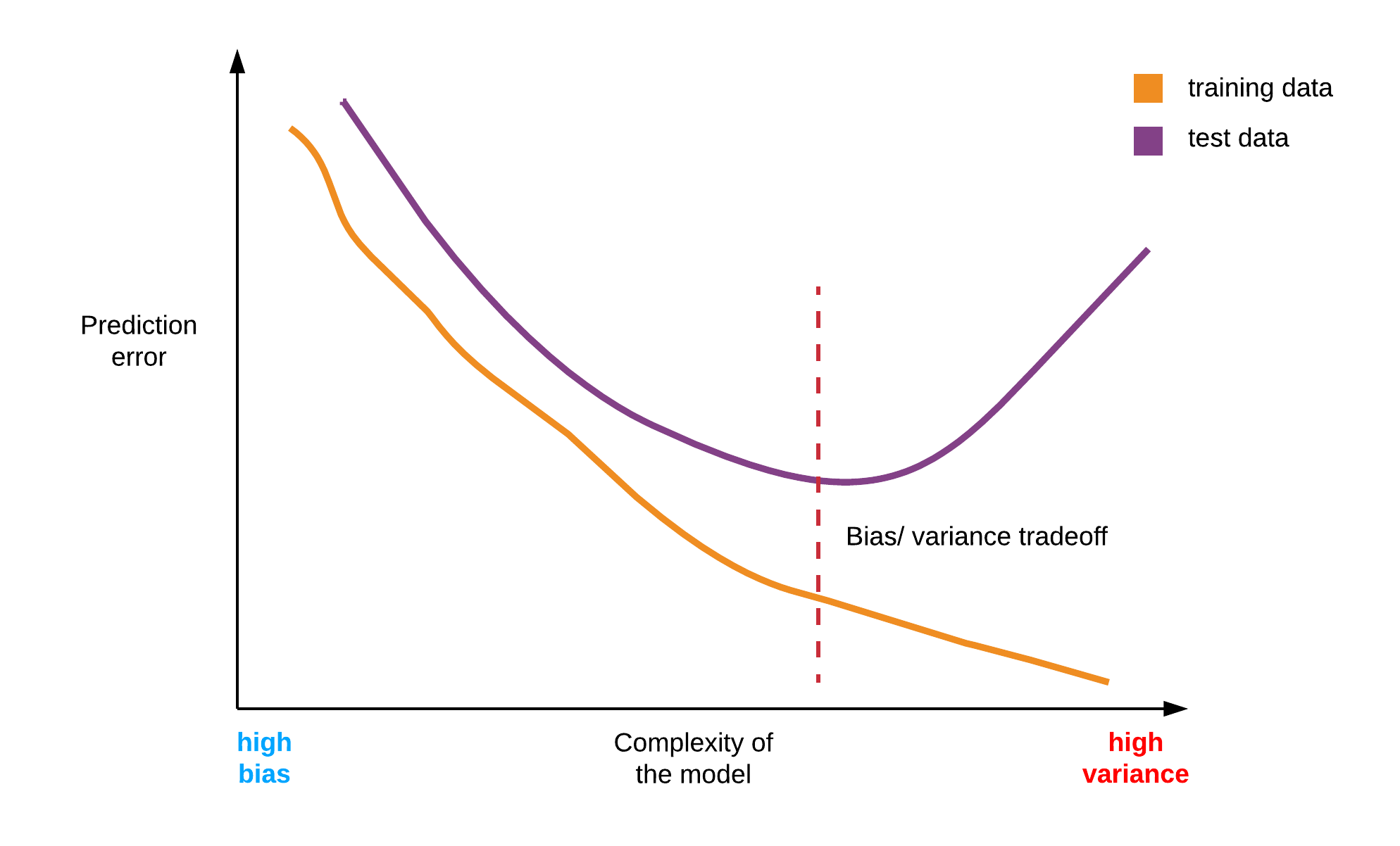The goal is to have a model that generalizes to new examples. Finding this middle ground is what is known as the bias/ variance tradeoff.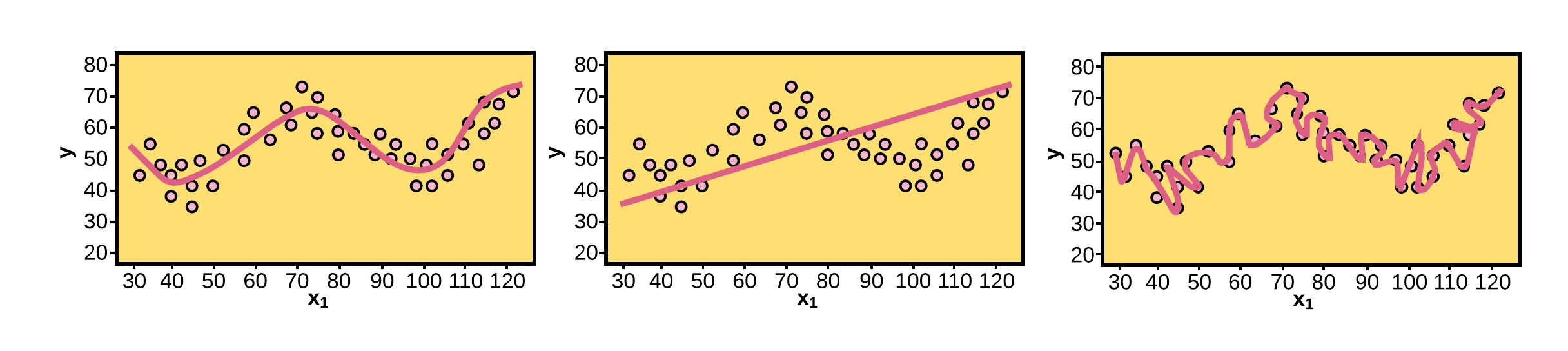## Evaluation metrics

Evaluation metrics give us a way to measure the performance of our model. Let’s see some common evaluation metrics.

Classification.

• Confusion matrix.
• AUC-ROC (Area under ROC curve).

Regression

• Root Mean Squared Error (RMSE).
• R-squared ( $R^2$ ).

### Confusion Matrix

Confusion matrix is one of the more popular metrics for assessing the performance of a classification supervised machine learning model.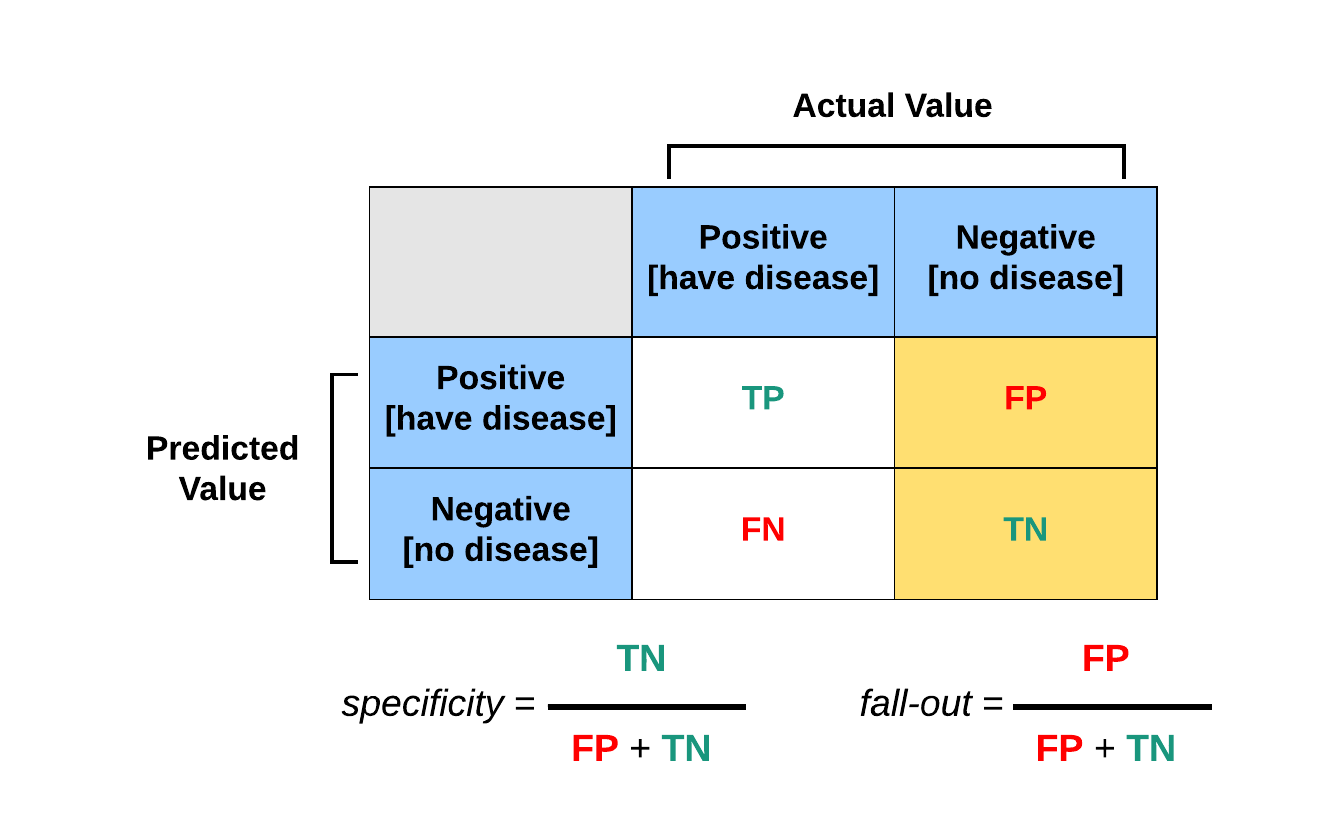### Root Mean Squared Error (RMSE)

• Root Mean Squared Error shortened for RMSE is an important evaluation metric in supervised machine learning for regression problems.
• The goal of RMSE is to calculate the error difference between the original targets and the predicted targets made by the learning algorithm.

\begin{equation} RMSE=\sqrt{\displaystyle \frac{ \sum_{i=1}^{n} {(y_{i}-{\hat{y}}_{i})^{2}} } {n} }. \end{equation}

### Resampling

Resampling is another important concept for evaluating the performance of a supervised learning algorithm and it involves selecting a subset of the available dataset, training on that data subset and using the reminder of the data to evaluate the trained model. Methods for resampling include:

• The validation set technique,
• The Leave-one-out cross-validation technique (LOOCV), and
• The k-fold cross-validation technique.

Validation set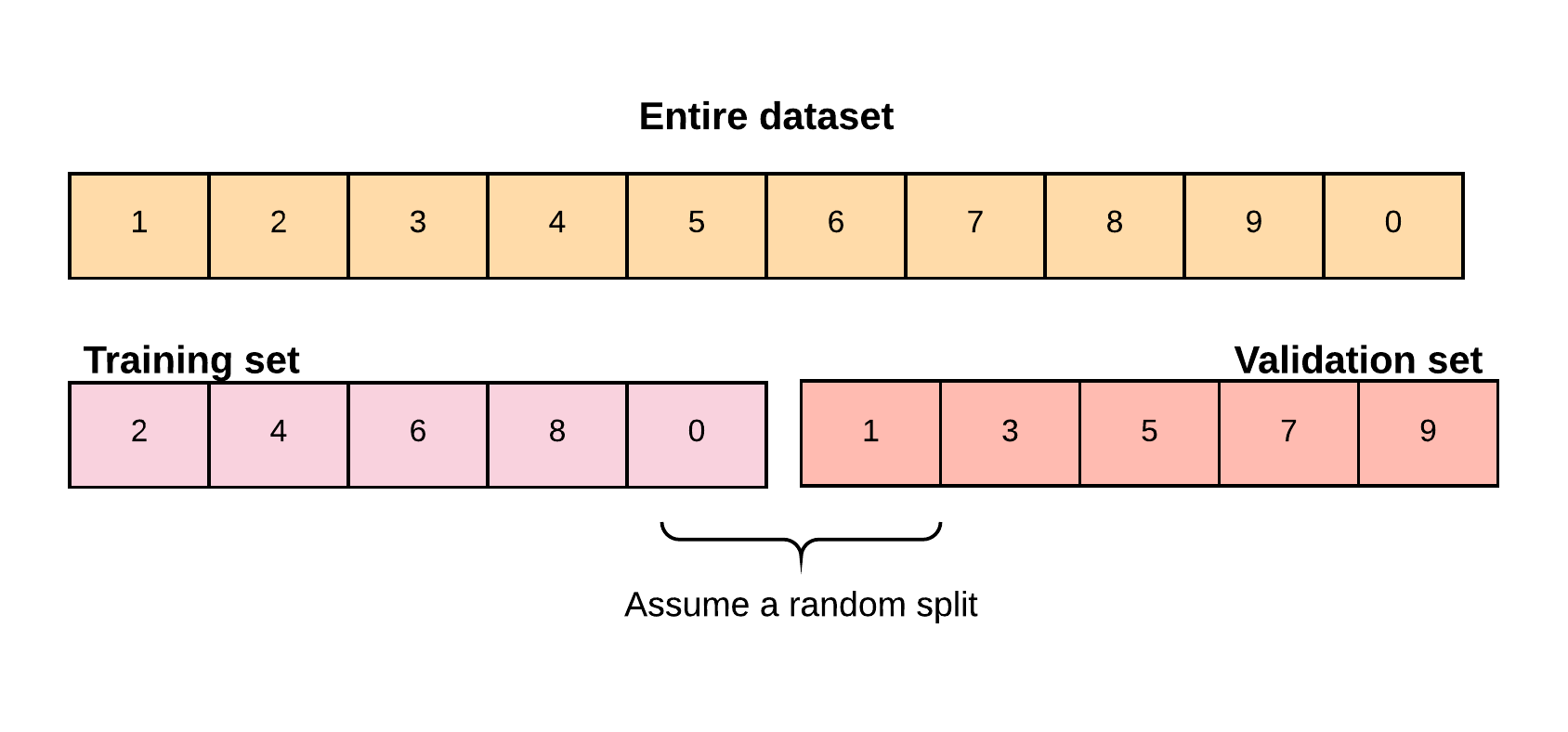k-Fold validation